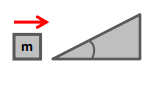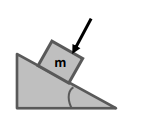Ch 07: Friction, Inclines, SystemsWorksheetSee all chapters
 Ch 01: Intro to Physics; Units 1hr & 22mins 0% complete Worksheet Ch 02: 1D Motion / Kinematics 4hrs & 13mins 0% complete Worksheet Ch 03: Vectors 2hrs & 2mins 0% complete Worksheet Ch 04: 2D Kinematics 2hrs 0% complete Worksheet Ch 05: Projectile Motion 2hrs & 57mins 0% complete Worksheet Ch 06: Intro to Forces (Dynamics) 3hrs & 42mins 0% complete Worksheet Ch 07: Friction, Inclines, Systems 0% complete Worksheet Ch 08: Centripetal Forces & Gravitation 3hrs & 51mins 0% complete Worksheet Ch 09: Work & Energy 3hrs & 56mins 0% complete Worksheet Ch 10: Conservation of Energy 7hrs & 4mins 0% complete Worksheet Ch 11: Momentum & Impulse 5hrs & 35mins 0% complete Worksheet Ch 12: Rotational Kinematics 3hrs & 4mins 0% complete Worksheet Ch 13: Rotational Inertia & Energy 7hrs & 7mins 0% complete Worksheet Ch 14: Torque & Rotational Dynamics 2hrs & 9mins 0% complete Worksheet Ch 15: Rotational Equilibrium 4hrs & 10mins 0% complete Worksheet Ch 16: Angular Momentum 3hrs & 6mins 0% complete Worksheet Ch 17: Periodic Motion 2hrs & 17mins 0% complete Worksheet Ch 19: Waves & Sound 3hrs & 25mins 0% complete Worksheet Ch 20: Fluid Mechanics 4hrs & 39mins 0% complete Worksheet Ch 21: Heat and Temperature 4hrs & 9mins 0% complete Worksheet Ch 22: Kinetic Theory of Ideal Gasses 1hr & 40mins 0% complete Worksheet Ch 23: The First Law of Thermodynamics 1hr & 49mins 0% complete Worksheet Ch 24: The Second Law of Thermodynamics 4hrs & 56mins 0% complete Worksheet Ch 25: Electric Force & Field; Gauss' Law 3hrs & 32mins 0% complete Worksheet Ch 26: Electric Potential 1hr & 55mins 0% complete Worksheet Ch 27: Capacitors & Dielectrics 2hrs & 2mins 0% complete Worksheet Ch 28: Resistors & DC Circuits 3hrs & 20mins 0% complete Worksheet Ch 29: Magnetic Fields and Forces 2hrs & 35mins 0% complete Worksheet Ch 30: Sources of Magnetic Field 2hrs & 30mins 0% complete Worksheet Ch 31: Induction and Inductance 3hrs & 38mins 0% complete Worksheet Ch 32: Alternating Current 2hrs & 37mins 0% complete Worksheet Ch 33: Electromagnetic Waves 1hr & 12mins 0% complete Worksheet Ch 34: Geometric Optics 3hrs 0% complete Worksheet Ch 35: Wave Optics 1hr & 15mins 0% complete Worksheet Ch 37: Special Relativity 2hrs & 10mins 0% complete Worksheet Ch 38: Particle-Wave Duality Not available yet Ch 39: Atomic Structure Not available yet Ch 40: Nuclear Physics Not available yet Ch 41: Quantum Mechanics Not available yet

# Inclined Planes with Friction

See all sections
Sections
Intro to Friction
Kinetic Friction
Static Friction
Inclined Planes
Inclined Planes with Friction
Forces in Connected Objects (Systems)
Intro to Springs (Hooke's Law)

Concept #1: Intro to Incline Plane with Friction

Transcript

Concept #2: Incline with Friction & Critical Angle

Transcript

Example #1: Incline with Friction

Transcript

Example #2: Incline with Friction

Transcript

Alright, so here we have a block it gets to the bottom of an inclined plane with 15 meters per second, so I'm going to say that the initial velocity at the bottom here is 15, the incline makes an angle of 25 degrees with the horizontal right there and the coefficients of friction are 0.4 so that's mu kinetic is 0.4 and mu static is 0.8, we want to know the block's acceleration so this implies it is the block's acceleration as it's going up the plane so the block's going to move up the plane, and it eventually stops because it's going against gravity and it's going against kinetic friction since it's moving so it eventually going to stop so the initial velocity here is 15 and the final velocity here is 0 if you wanted to draw a sketch of...not a full free body diagram but just a quick sketch of the forces you would have MG down here, you would have MGx is always down the plane the normal up the plane, MGy into the plane and you also have friction, you friction because of the coefficients of friction that is given to you which way do you think friction would be? And I hope you're thinking that since we're moving up I'm going to call it a positive direction that friction will be opposite to that and it is, it's the kinetic friction opposite to you and to find the acceleration we're simply going to use F=MA, sum of all forces along the X axis here is a MAx or simply just A, alright? And then the forces are as you're going up there's only two forces here in the X axis which are these two, a lot of people or a good amount of people get confused with questions like this and they think that since you're moving up the plane there has to be a force going up the plane and that's just wrong you don't need a force to be pushing you up the plane for you to move up the plane you go up the plane because you had an initial velocity and because of inertia you just keep going, right? It's because at some point before you got to the bottom of the plane somebody pushed you, you got 50 meters per second and then you go up the plane but there are no forces going up OK? One way that you might detect that is that if you think there's a force going up all the sudden you can't put a finger on it you can't figure out which force that is or what number magnitude that force has and that's because it doesn't exist, OK? So, the only two forces here and I'm going to say that the forces are where the forces are negative MGx and negative friction kinetic It's kinetic friction it's a rubbing friction because you're sliding the block is sliding this equals to MA, OK? We want to find acceleration here so what I'm going to do is I'm going to expand these, now one way you could do this you could calculate MGx calculate friction and just plug in the numbers then I'm going to expand this MG sine of theta minus friction now friction mu normal and in an inclined plane normal is the same as MGy so it's mu MGy and MGy is MG cosine of theta, right? You should memorize that MGy is MG cosine of theta if the angle is down here and little F becomes all of this OK, so minus mu MG cosine of theta and just to be completely clear here, this mu is mu kinetic and that equals to MA if you do this the masses will cancel which is and I can combine the Gs, OK? There's also two negatives here so you can factor the negative G sine of theta plus mu kinetic cosine of theta that is our acceleration OK? If you are having a hard time seeing how this goes from here to here you can work it out yourself slowly and see how these two are actually the same cool? So that's sort of the expression for I can plug in numbers or again you could have just found a calculator these individually and plug it in there maybe that would be a little bit easier whatever you like OK. So A equals negative 10 sine of 25, I have it here sine of 25 is 0.42 plus mu which is 0.4 because it's mu kinetic cosine of twenty five is 0.91 and I calculated this earlier it 7.84 meters per second squared and I got a negative and it should make sense that I get a negative because I said that this is the direction of positive right here up the plane and that's arbitrary I just said well if it's going up let's call that positive direction and then therefore the acceleration is going against you because you're slowing down so it's negative, OK that's it for part A. For Part B it says once you get to the highest point will it move back down? What happens at the highest point? Well at the highest point final over here at the highest point there are our velocity will be 0, OK? I'm going to say the V top is 0 question is will it move back down? In other words, is there going to be an acceleration but instead of calculating the acceleration we just want to yes or no question and basically the idea is are there enough forces or is there enough force pulling it down to overcome static friction once you get to the top as you're moving up you're going against kinetic friction back down this way, OK? So, as you're going up you have kinetic friction once you get to the top and you stop V equals 0 now what do you have? you have an MGx pulling you down and you have static friction this way. Remember friction is almost always Anyway friction is almost always opposing motion and if the box stops again this a box it's not a ball, so let's draw a little box if the box stops at the top in now switches direction this way so friction is going to going to go this way and it's going to try to keep it from moving and to figure out whether or not it moves we have to compare the forces, OK? I can calculate MGx If you had already calculated it here it's the same number but if you calculate MGx you get the mass is 10 gravity is 10 and then it is the sine of 25 and if you do this you get a 42.3 OK? all we're doing is comparing the forces, friction static is mu static normal mu static is 0.8, normal is right here all of this here is normal MG cosine of theta so MG cosine of 25 and again feel free to solve this as slowly as you have to and if you do those the answer is 72.5 Newtons, so this block will not move down, OK? I'm going to write here since MGx is less than the static friction (the maximum static friction) the acceleration is 0 so the block does not move let me disappear here the block does not move so the answer's no it doesn't move, OK? So that's interesting as are going up the plane as you're going up the plane there is kinetic friction pulling down the second you get to the top it switches to static friction because for an instant of the second you're not moving and then you actually stay there and you don't fall because static friction is too big and MGx is not able to overcome it, alright? So that's it for this one hopefully you'll make sense let me know if you have any questions.

Practice: A 5 kg block reaches the bottom of a long inclined plane with 20 m/s. The incline makes 53° with the horizontal, and the block-incline coefficient of friction is 0.4. What total time does it take the block to go up and back down?Practice: A 10 kg block is pushed against an inclined plane with a force F that is perpendicular to the plane, as shown. The incline makes an angle of 53° with the horizontal, and the coefficients of friction between the block and the incline are 0.5 and 0.6. What minimum force F is needed to keep the block from sliding down the incline?